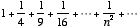index: click on a letter A B C D E F G H I J K L M N O P Q R S T U V W X Y Z A to Z index index: subject areas numbers & symbols sets, logic, proofs geometry algebra trigonometry advanced algebra & pre-calculus calculus advanced topics probability & statistics real world applications multimedia entrieswww.mathwords.com about mathwords website feedback

 Infinite Series A series that has no last term, such as. The sum of an infinite series is defined as the limit of the sequence of partial sums. Note: The infinite series above happens to have a sum of π2/6.See also